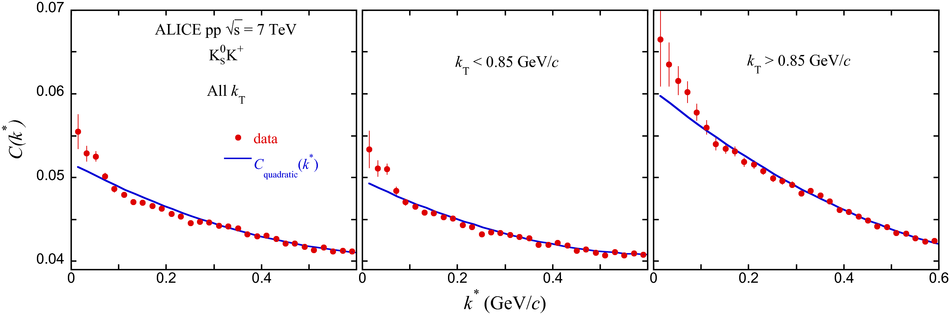# Figure 4

 Sample raw correlation functions for K$^0_{\rm S}$K$^+$ showing the fitted quadratic baseline function, Eq.~\ref{quad} Statistical uncertainties are shown. The scale of $C(k^*)$ is arbitrary.